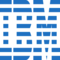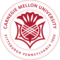How to Improve Accuracy of Logistic Regression

# How to Improve Accuracy of Logistic Regression

clickHere
Updated on Jan 31, 2023 11:02 IST

## Introduction

Everyone wants their model to yield 100 percent accuracy, but, almost 80 percent of the time is spent is cleaning just to attain 80-90 percent of accuracy! why is that so? It can be due to multiple reasons, for example, clumsy data, unformatted data, etc. but still, people worry about achieving the remaining 20 percent accuracy so that they can simply satisfy their client or just brag about their accuracy!! In this blog, we will be talking about Logistic Regression

In order to improve model accuracy, you need to focus on a few things like feature scaling, hyper-parameter tuning, etc! So, without making any delay let’s jump into the article

## What is Logistic Regression?

The supervised machine learning method Logistic Regression is used to predict outcomes. The main aim of logistic regression is to predict a query sample’s classification (e.g., yes/no). With the help of a sigmoid function, it estimates the probability of the action (between 0 and 1) using labeled input data. A threshold value is chosen as a cut-off for an event projected to occur in order to determine the class outcome

Now, let us see how we can build and tune the Logistic Regression model!

Before Jumping into that, I just give you a brief introduction to the dataset used in this example.

## Description of the Dataset:

The data used here is Mammographic data, Breast cancer screening with mammography is the most effective approach available today. However, because of the low positive predictive value of breast biopsy as a result of mammography interpretation, over 70% of needless biopsies with benign outcomes are performed. Several computer-aided diagnostic (CAD) solutions have been proposed in recent years to reduce the large incidence of needless breast biopsies. These systems aid clinicians in deciding whether to perform a breast biopsy on a worrisome lesion identified on a mammogram or instead undertake a short-term follow-up examination

From BI-RADS attributes and the patient’s age, this data set can be utilized to estimate the severity (benign or malignant) of a mammographic mass lesion. It contains a BI-RADS assessment, the patient’s age, and three BI-RADS attributes, as well as the ground truth (the severity field), for 516 benign and 445 malignant masses identified on full-field digital mammograms collected at the University Erlangen-Institute Nuremberg’s of Radiology between 2003 and 2006.

In a double-review procedure, clinicians provide a BI-RADS score for each incident, ranging from 1 (certainly benign) to 5 (strongly suggestive of cancer). Sensitivities and associated specificities can be estimated if all instances with BI-RADS assessments greater than or equal to a given value (ranging from 1 to 5) are malignant and the remaining cases are benign. These can be used to determine how well a CAD system performs in comparison to radiologists.

### Attribute Information:

1. Age: patient’s age in years (integer)
2. Shape: mass shape: round=1 oval=2 lobular=3 irregular=4 (nominal)
3. Margin: mass margin: circumscribed=1 micro lobulated=2 obscured=3 ill-defined=4 spiculated=5 (nominal)
4. Density: mass density high=1 iso=2 low=3 fat-containing=4 (ordinal)
5. Severity: benign=0 or malignant=1 (binominal)

Now that we know all the necessary information about the data, let’s start building our model, before moving! the data is mostly cleaned hence I won’t be spending much time in the cleaning process! Instead, we will focus on how to do the feature scaling, hyperparameter tuning, etc

## Understanding Logistic Regression with an Example

As always let us import the libraries

` `
`#required for mathematical operationsimport numpy as npimport pandas as pd #required for feature scalingfrom sklearn.preprocessing import StandardScaler #required for splitting the datafrom sklearn.model_selection import train_test_split #required for building the modelfrom sklearn.linear_model import LogisticRegression #required to evaluate the resultfrom sklearn.metrics import classification_report #required for grahical representation purposefrom sklearn.metrics import roc_curve, aucimport matplotlib.pyplot as pltimport seaborn as sns %matplotlib inline #required for parameter tuningfrom sklearn.linear_model import LogisticRegressionfrom sklearn.model_selection import GridSearchCVCopy code`

Let us import the data and see the sample of it

` `
`data = pd.read_csv('/content/Mamographic.csv')data.head()Copy code`

let us check the shape of the data, as to how many rows and columns does our data have

` `
`data.shapeCopy code`

Now check the data type, null values (if any) present in our data or not

` `
`data.info()Copy code`

Now, in order to improve our model’s accuracy, let us perform feature scaling on SHAPE, MARGIN, and DENSITY Features by converting them into objects because they are nominal as mentioned in the dataset description

` `
`# converting data type for SHAPEdata['SHAPE'] = data['SHAPE'].astype(str)# converting data type for MARGINdata['MARGIN'] = data['MARGIN'].astype(str)# converting data type for DENSITYdata['DENSITY'] = data['DENSITY'].astype(str)Copy code`

Let us Divide dependent and independent variables, the purpose is to predict the dependent variable using the independent variables (or characteristics) (or outcome). As a result, these variables must be divided into X and y, with X representing all the features entered the model and y representing the model’s eventual result

` `
`#dropping out SEVERITY column as that is the dependent oneX = data.drop('SEVERITY',axis=1) y = data.SEVERITY #printing the shapes of X and y print("shape of X is :",X.shape)print("shape of y is :",y.shape)Copy code`

Let us encode the independent variables

` `
`X= pd.get_dummies(X)X.shapeCopy code`

Notice how the number of X.shape characteristics has increased from four to fourteen. This indicates that the model now has ten extra features. Refer to the following code to learn more about the new features.

` `
`feature = X.columns.tolist()print(feature)Copy code`

For example, You can notice that MARGIN is broken down into MARGIN_1, MARGIN_2, MARGIN_3, MARGIN_4, MARGIN_5

MARGIN_1 is represented as [1, 0 , 0, 0, 0]

MARGIN_2 is represented as [0, 1 , 0, 0, 0]

MARGIN_3 is represented as [0, 0 , 1, 0, 0]

MARGIN_4 is represented as [0, 0 , 0, 1, 0]

MARGIN_5 is represented as [0, 0 , 0, 0, 1]

This is basically one_hot_encoding where each label is mapped into a binary vector

We performed this step because Input and output variables must be represented as numbers in machine learning algorithms. Because this data set contains categorical features, they must be converted to integers before fitting and evaluating a model

We perform feature scaling because The Euclidean distance is used by some machine learning algorithms to calculate the distance between two points. If one of the features has a wide range of values, it will be dominant in determining the distance. Standardization and normalizing are approaches that are applied to a set of independent variables to ensure that each feature contributes proportionately to the final distance.

` `
`standared_scaler = StandardScaler()X = standared_scaler.fit_transform(X)print(X)Copy code`

Now, we are going to split the data into training and testing! where training is used to train, model or fit the data but testing data is used to obtain the unbiased result for the final model.

` `
`X_train, X_test, y_train, y_test = train_test_split(X, y, test_size = 0.3)Copy code`

It is critical to have an unbiased review to assess the model’s predicted performance. Splitting the dataset before utilizing it is one way to accomplish this. The data are randomly divided into two sets: a training set and a testing set, with 70 percent of the data put aside for training and the remaining 30 percent set away for testing.

In this step, we will use training data to train the model so that it can properly predict the outcome.

` `
`logistic_Model = LogisticRegression(random_state=1234)logistic_Model.fit(X_train, y_train)Copy code`

Here we will evaluate the model’s correctness and efficiency by obtaining model predictions on testing data.

` `
`y_predicted = logistic_Model.predict(X_test)print(y_predicted)Copy code`

Now, we shall evaluate the classification model

` `
`print("Classification Report is: \n",classification_report(y_test, y_predicted))Copy code`

Here are a few metrics in the classification reports namely,

accuracy, precision, recall, f1 score

Now with the help of ROC and AUC curve, let us just plot the area please add one line definition for AUC and roc curve

` `
`probability               = logistic_Model.predict_proba(X_test)predicatbility               = probability[:,1]false_positive_rate, true_positive_rate, threshold = roc_curve(y_test, predicatbility)roc_auc             = auc(false_positive_rate, true_positive_rate) plt.title('ROC')plt.plot(false_positive_rate, true_positive_rate, 'red', label = 'AUC = %0.3f' % roc_auc)plt.legend(loc = 'upper left')plt.plot([0, 1], [0, 1],'b--')plt.xlim([0, 1])plt.ylim([0, 1])plt.ylabel('True Positive Rate')plt.xlabel('False Positive Rate')plt.show()Copy code`

Let us perform parameter tuning now, Basically, Parameter tuning is performed to choose the parameters that will be utilized to find the best combination.

` `
`parameter_grid_logistic_regression = {    'max_iter': [20, 50, 100, 200, 500, 1000],                      # Number of iterations    'solver': ['newton-cg', 'lbfgs', 'liblinear', 'sag', 'saga'],   # Algorithm to use for optimization    'class_weight': ['balanced']                                    # Troubleshoot unbalanced data sampling}Copy code`

where,

max_iter is the number of iterations

solver is the algorithm that we use for optimization

class_weight is used to troubleshoot the imbalance of data sampling

Now, to improve results, we shall discover the best combination of hyperparameters that minimizes a predetermined loss function.

` `
`logistic_Model_grid = GridSearchCV(estimator=LogisticRegression(random_state=1234), param_grid=parameter_grid_logistic_regression, verbose=1,                     cv=10, n_jobs=-1) logistic_Model_grid.fit(X_train, y_train) print("Best score for the model after tuning is: ",logistic_Model_grid.best_score_)print("Best parameters for the model is :",logistic_Model_grid.best_estimator_)Copy code`

You must note that here,

The cv is defined as 10 and there are 30 candidates, the total number of fits is 300 (max iter has 6 defined parameters, the solver has 5 defined parameters, and class weight has 1 defined parameter). As a result, the total number of fits is calculated as 10 x [6 x 5 x 1] = 300

the estimator is the machine learning model of interest, assuming it contains a scoring function; in this example, the model is LogisticRegression ()

random_state is the seed of the pseudo-random number generator to utilize while shuffling the data in the random state. Set the seed to a consistent number for model-to-model comparison to avoid deviations in model numeric evaluation output; in this case, the value is 1234

param_grid is a dictionary that has parameter names (strings) as keys and lists of parameter settings to attempt as values, allowing you to search through any sequence of parameter settings

verbose is the verbosity, if a higher number is assigned higher messages are shown, here it is set just to 1

Now, that we have got the best parameters and the best score, you can implement this criterion and train your model again for better accuracy! As our model has good accuracy before itself, I am not going to train again and overfit the model!

## Conclusion

Hope this article helps you to improve the accuracy of the Logistic model by incorporating different methods as mentioned above!!

If you are interested in going into the data science field then you find different study material on this page.

clickHere

This is a collection of insightful articles from domain experts in the fields of Cloud Computing, DevOps, AWS, Data Science, Machine Learning, AI, and Natural Language Processing. The range of topics caters to upski... Read Full Bio

## Trending Data Science CoursesMachine Learning
Coursera4.6Calculus for Machine Learning and Data Science
Coursera4.8## Top Picks & New Arrivals